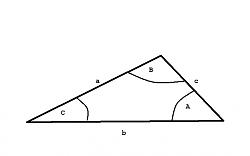Free 173 Best Homemade Tools eBook:

# Thread: How to solve any triangle

1. ## How to solve any triangle

Trigonometry is all about triangles - and not just right triangles. This discussion will show you how to solve plane triangles of any type.

Any triangle has six items of information - three sides (a,b,c) and the angles opposite those sides (A,B,C). In order to solve a triangle you need to know three of those items AND AT LEAST ONE OF THEM MUST BE A SIDE. (Here "solve" means find all the unknown items.)

In the discussion that follows we will be making use of three relations that are true for ANY triangle.

Relation 1: The angles in a triangle always sum to 180 degrees.

A + B + C = 180

Relation 2: The law of cosines.

a^2 = b^2 + c^2 - 2bc * cos (A)
b^2 = a^2 + c^2 - 2ac * cos (B)
c^2 = a^2 + b^2 - 2ab * cos (C)

Aside: Note that if one of the angles is 90 degrees, cos(90) = 0 and the law of cosines reduces to the Pythagorean theorem.

Relation 3: The law of sines

sin(A) / a = sin(B) / b = sin(C) / c

There are five possible combinations for the three known items. [The triples in brackets show an example.]

SAS - Two adjacent sides and the angle between them are known [a,C,b]
SSA - Two sides and the angle opposite one of the sides is known [a,b,A]
ASA - A side and the two angles to which it connects are known [B,a,C]
AAS - Two angles and the side opposite one of the angles is known [A,B,a]
SSS - All three sides are known [a,b,c]

The technique for solving each of these possible combinations is shown; including an example using the knowns indicated in the bracketed triples above.

SAS [a,C,b]

Use the law of cosines to find the unknown side: c^2 = a^2 + b^2 - 2ab * cos (C)
Use the law of sines to find one of the unknown angles: A = arcsin [a * sin(C) / c]
Find the third angle: B = 180 - A - C

SSA [a,b,A]

Use the law of sines to find one of the unknown angles: B = arcsin [b * sin(A) / a]
Find the third angle: C = 180 - A - B
Use the law of cosines to find the unknown side: c^2 = a^2 + b^2 - 2ab * cos (C)

ASA [B,a,C]

Find the third angle: A = 180 - B - C
Use the law of sines to find the unknown sides: b = a * sin(B) / sin (A) and c = a * sin(C) / sin (A)

AAS [A,B,a]

Find the third angle: C = 180 - A - B
Use the law of sines to find the unknown sides: b = a * sin(B) / sin (A) and c = a * sin(C) / sin (A)

SSS - [a,b,c]

Use the law of cosines to find one of the angles: A = arccos[(b^2 + c^2 - a^2) / (2bc)]
Use the law of sines to find one of the unknown angles: B = arcsin [b * sin(A) / a] [could also use law of cosines]
Find the third angle: C = 180 - A - B

After you've solved your triangle, check your work. The angles should add to 180 degrees and the sides should satisfy the law of sines.
The procedures outlined here are implemented in the TRIANGLE program available on my website.Reply With Quote

2. ## The Following 9 Users Say Thank You to mklotz For This Useful Post:

DIYSwede (Apr 28, 2021), hegefer (Apr 27, 2021), Jon (Apr 30, 2021), Moby Duck (Apr 28, 2021), Ralphxyz (Apr 28, 2021), RetiredFAE (Apr 27, 2021), rgsparber (Apr 27, 2021), sacco1 (May 2, 2021), Tuomas (Apr 28, 2021)

3. I always did ok in math until the alphabet got involved. Now it just doesnt seem that important anymore.🤣🤣Reply With Quote

4.Originally Posted by stillldoinitI always did ok in math until the alphabet got involved. Now it just doesn’t seem that important anymore.����
I presume that your "alphabet" is a reference to algebra. Algebra is the language of mathematics; you can't do much beyond arithmetic without developing some facility with algebra. Take the time to learn it; mathematics is a most useful tool in the shop.

Once you understand that quantities can be represented by symbols (usually letters of several alphabets) and that the symbols can be manipulated in the same way that one might manipulate the quantities themselves, algebra becomes intuitive.Reply With Quote